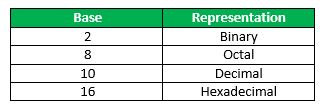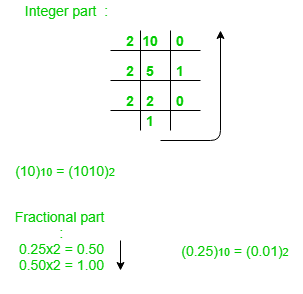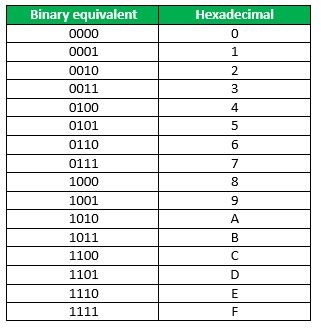Related Articles

# Number System and Base Conversions

• Difficulty Level : Easy
• Last Updated : 07 Jul, 2021

Electronic and Digital systems may use a variety of different number systems, (e.g. Decimal, Hexadecimal, Octal, Binary).

A number N in base or radix b can be written as:

`(N)b = dn-1 dn-2 -- -- -- -- d1 d0 . d-1 d-2 -- -- -- -- d-m`

In the above, dn-1 to d0 is the integer part, then follows a radix point, and then d-1 to d-m is the fractional part.

dn-1 = Most significant bit (MSB)
d-m = Least significant bit (LSB)How to convert a number from one base to another?

## 1. Decimal to Binary

(10.25)10Note: Keep multiplying the fractional part with 2 until decimal part 0.00 is obtained.
(0.25)10 = (0.01)2

## 2. Binary to Decimal

```(1010.01)2
1x23 + 0x22 + 1x21+ 0x20 + 0x2 -1 + 1x2 -2 = 8+0+2+0+0+0.25 = 10.25
(1010.01)2 = (10.25)10 ```

## 3. Decimal to Octal

```(10.25)10
(10)10 = (12)8
Fractional part:
0.25 x 8 = 2.00 ```

Note: Keep multiplying the fractional part with 8 until decimal part .00 is obtained.
(.25)10 = (.2)8

## 4. Octal to Decimal

```(12.2)8
1 x 81 + 2 x 80 +2 x 8-1 = 8+2+0.25 = 10.25
(12.2)8 = (10.25)10 ```

To convert from Hexadecimal to Binary, write the 4-bit binary equivalent of hexadecimal.(3A)16 = (00111010)2

To convert from Binary to Hexadecimal, start grouping the bits in groups of 4 from the right-end and write the equivalent hexadecimal for the 4-bit binary. Add extra 0’s on the left to adjust the groups.

```1111011011
0011 1101 1011
(001111011011 )2 = (3DB)16 ```